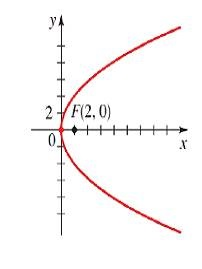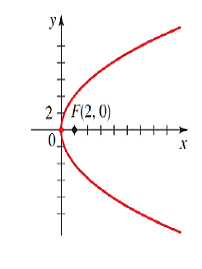Chapter 12.CR, Problem 37E### Algebra and Trigonometry (MindTap ...

4th Edition
James Stewart + 2 others
ISBN: 9781305071742

#### Solutions

Chapter
Section### Algebra and Trigonometry (MindTap ...

4th Edition
James Stewart + 2 others
ISBN: 9781305071742
Textbook Problem

# 37-42 Finding the Equation of a Conic Find an equation for the conic whose graph is shown.To determine

To find:

An equation of the conic.Explanation

Given:

The foci is F(2,0).

Approach:

An equation of right handed parabola is y2=4px

Calculation:

The foci is F(2,0) implies p=2

The foci are on the positive <

### Still sussing out bartleby?

Check out a sample textbook solution.

See a sample solution

#### The Solution to Your Study Problems

Bartleby provides explanations to thousands of textbook problems written by our experts, many with advanced degrees!

Get Started